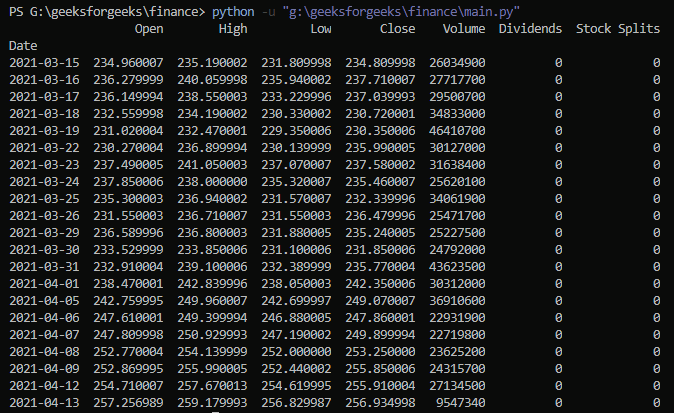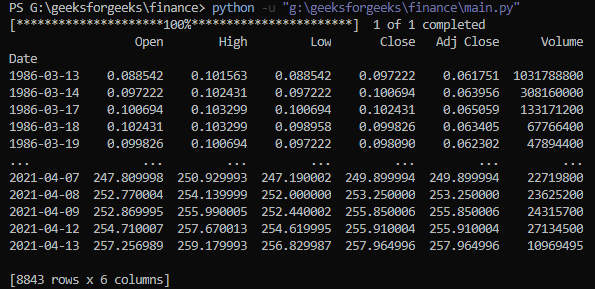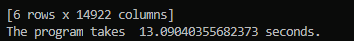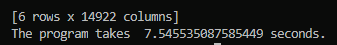• Last Updated : 15 Sep, 2021

Yfinance is a python package that enables us to fetch historical market data from Yahoo Finance API in a Pythonic way. It becomes so easy for all the Python developers to get data with the help of yfinance.

We can easily download historical stock data from yfinance, but the problem is, it is very time taking. Hence, we use multithreading for covering up the time. Multithreading enables us to download large amounts of data by executing multiple threads concurrently.

## Installation

This module does not come built-in with Python. To install it type the below command in the terminal.

`pip install yfinance`

Let’s see the process step by step which are explained below with the implementation:

Step 1: Get all the required modules

## Python3

 `import` `yfinance as yf`

Step 2: Getting stocks history data

## Python

 `# Get the stocks info``import` `yfinance as yf` `# Ticker is a function responsible``# for fetching the data MSFT is``# representing info about Microsoft``# Corporation``msft ``=` `yf.Ticker(``'MSFT'``)` `# msft.info will return all information``# about microsoft corporation``data ``=` `msft.history()` `# printing the data``print``(data)`

Output:This is a recent year’s stock data of Microsoft Corporation. Please note that if we use data = msft.history(period=’max’) it will return all data till now.

## Python3

 `# pip install yfinance``import` `yfinance as yf` `# Here we use yf.download function``data ``=` `yf.download(` `    ``# tickers has value of company``    ``# shortname``    ``tickers``=``'MSFT'``,``)` `# printing the data``print``(data)`

Output:Hence, 1 of 1 download is completed.  It is all data from beginning to end till now of only one company.

This is a code for downloading the data from companies like IBM, Apple (AAPL), Amazon(AMZN), Microsoft(MSFT), etc.

## Python3

 `# pip install yfinance``import` `yfinance as yf` `ticker_list ``=` `[``'IBM'``, ``'MSFT'``, ``'AAPL'``, ``'AMZN'``]` `# Here we use yf.download function``data ``=` `yf.download(``    ` `    ``# passes the ticker``    ``tickers``=``ticker_list,``    ` `    ``# used for access data[ticker]``    ``group_by``=``'ticker'``,` `)` `# used for making transpose``data ``=` `data.T` `for` `t ``in` `ticker_list:``  ` `    ``# printing name``    ``print``(t)``    ``print``(``'\n'``)``    ` `    ``# used data.loc as it takes only index``    ``# labels and returns dataframe``    ``print``(data.loc[t]) ``    ``print``(``'\n'``)`

Output:Step 5: Calculating the time of execution and using multithreading

tickers=ticker_list,

group_by=’ticker’,

)

Program for calculating time, we use the time module

## Python

 `# pip install yfinance``import` `yfinance as yf``import` `time` `# Time starts from here``start ``=` `time.time()` `ticker_list ``=` `[``'IBM'``, ``'MSFT'``, ``'AAPL'``, ``'AMZN'``]` `# Here we use yf.download function``data ``=` `yf.download(``    ``tickers``=``ticker_list,``    ``threads``=``True``,``    ``group_by``=``'ticker'``,` `)` `# used for making transpose``data ``=` `data.T` `for` `t ``in` `ticker_list:``    ``print``(t)``    ` `    ``# used data.loc as it takes only index``    ``# labels and returns dataframe``    ``print``(data.loc[t]) ` `# Total time calculated``print``(``'The program takes '``, time.time()``-``start, ``'seconds.'``)`